AP State Board Syllabus AP SSC 10th Class Maths Textbook Solutions Chapter 11 Trigonometry Ex 11.2 Textbook Questions and Answers.

## AP State Syllabus SSC 10th Class Maths Solutions 11th Lesson Trigonometry Exercise 11.2

### 10th Class Maths 11th Lesson Trigonometry Ex 11.2 Textbook Questions and Answers

Question 1.
Evaluate the following.
i) sin 45° + cos 45°
sin 45° + cos 45°
= $$\frac{1}{\sqrt{2}}$$ + $$\frac{1}{\sqrt{2}}$$
= $$\frac{1+1}{\sqrt{2}}$$
= $$\frac{2}{\sqrt{2}}$$
= $$\frac{\sqrt{2} \times \sqrt{2}}{\sqrt{2}}$$
= √2

ii)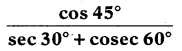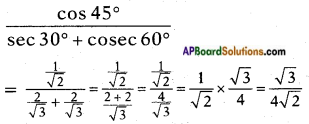iii)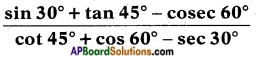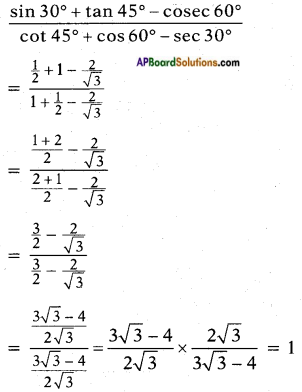iv) 2 tan2 45° + cos2 30° – sin2 60°
2 tan2 45° + cos2 30° – sin2 60°
= 2(1)2 + $$\left(\frac{\sqrt{3}}{2}\right)^{2}$$ – $$\left(\frac{\sqrt{3}}{2}\right)^{2}$$
= $$\frac{2}{1}$$ + $$\frac{3}{4}$$ – $$\frac{3}{4}$$
= $$\frac{8+3-3}{4}$$
= $$\frac{8}{4}$$
= 2v)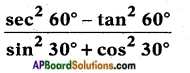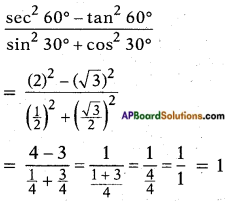Question 2.
Choose the right option and justify your choice.
i) $$\frac{2 \tan 30^{\circ}}{1+\tan ^{2} 45^{\circ}}$$
a) sin 60°
b) cos 60°
c) tan 30°
d) sin 30°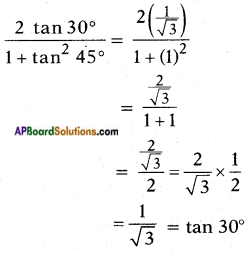ii) $$\frac{1-\tan ^{2} 45^{\circ}}{1+\tan ^{2} 45^{\circ}}$$
a) tan 90°
b) 1
c) sin 45°
d) 0
$$\frac{1-\tan ^{2} 45^{\circ}}{1+\tan ^{2} 45^{\circ}}$$ = $$\frac{1-(1)^{2}}{1+(1)^{2}}$$
= $$\frac{0}{1+1}$$ = $$\frac{0}{2}$$ = 0iii) $$\frac{2 \tan 30^{\circ}}{1-\tan ^{2} 30^{\circ}}$$
a) cos 60°
b) sin 60°
c) tan 60°
d) sin 30°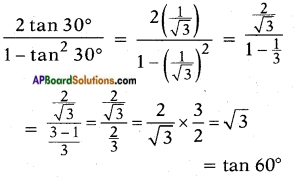Question 3.
Evaluate sin 60° cos 30° + sin 30° cos 60°. What is the value of sin (60° + 30°). What can you conclude?
Take sin 60°.cos 30° + sin 30°.cos 60°
= $$\frac{\sqrt{3}}{2}$$ . $$\frac{\sqrt{3}}{2}$$ + $$\frac{1}{2}$$ . $$\frac{1}{2}$$
= $$\frac{(\sqrt{3})^{2}}{4}$$ + $$\frac{1}{4}$$
= $$\frac{3}{4}$$ + $$\frac{1}{4}$$
= $$\frac{3+1}{4}$$
= $$\frac{4}{4}$$ = 1 …… (1)
Now take sin (60° + 30°)
= sin 90° = 1 …….. (2)
From equations (1) and (2), I conclude that
sin (60°+30°) = sin 60° . cos 30° + sin 30° . cos 60°.
i.e., sin (A + B) = sin A . cos B + cos A . sin B

Question 4.
Is it right to say cos (60° + 30°) = cos 60° cos 30° – sin 60° sin 30° ?
L.H.S. = cos (60° + 30°)
cos 90° = 0
R.H.S. = cos 60° . cos 30° – sin 60° . sin 30°.
= $$\frac{1}{2}$$ . $$\frac{\sqrt{3}}{2}$$ – $$\frac{\sqrt{3}}{2}$$ . $$\frac{1}{2}$$
= $$\frac{\sqrt{3}}{4}$$ – $$\frac{\sqrt{3}}{4}$$ = 0
∴ L.H.S = R.H.S
Yes, it is right to say
cos (60°+30°) = cos 60° . cos 30° – sin 60° . sin 30°.
i.e., cos (A + B) = cos A . cos B – sin A . sin BQuestion 5.
In right angle triangle △PQR, right angle is at Q and PQ = 6 cms, ∠RPQ = 60°. Determine the lengths of QR and PR.
Given that △PQR is a right angled triangle, right angle is at Q and PQ = 6 cm, ∠RPQ = 60°.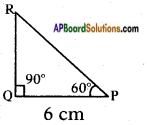tan 60° = $$\frac{\text { Opposite side to } \angle P}{\text { Adjacent side to } \angle P}$$
√3 = $$\frac{RQ}{6}$$
which gives RQ = 6√3 cm ……. (1)
To find the length of the side RQ, we consider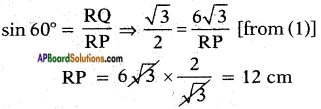∴ The length of QR is 6√3 and RP is 12 cm.

Question 6.
In △XYZ, right angle is at Y, YZ = x, and XY = 2x then determine ∠YXZ and ∠YZX.
Note: In the problem take
YX = x, and XZ = 2x.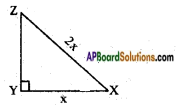Given that △XYZ is a right angled triangle and right angle at Y, and YX = x and XZ = 2x.
By Pythagoras theorem
XZ2 = XY2 + YZ2
(2x)2 = (x)2 + YZ2
4x2 = x2 + YZ2
YZ2 = 4x2 – x2 = 3x2
YZ = $$\sqrt{3 x^{2}}$$ = √3x
Now, from the △XYZ
tan X = $$\frac{XZ}{XY}$$ = $$\frac{\sqrt{3} x}{x}$$
tan X = √3 = tan 60°
∴ Angle YXZ is 60°.
tan Z = $$\frac{XY}{YZ}$$ = $$\frac{x}{\sqrt{3} x}$$
tan Z = $$\frac{1}{\sqrt{3}}$$ = tan 30°
∴ Angle YZX is 30°.
Hence ∠YXZ and ∠YZX are 60° and 30°.Question 7.
Is it right to say that
sin (A + B) = sin A + sin B? Justify your answer.
= $$\frac{1}{2}$$ + $$\frac{\sqrt{3}}{2}$$
= $$\frac{\sqrt{3}+1}{2}$$